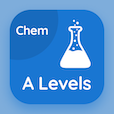# Business Mathematics Quiz PDF Online

Business Mathematics quiz questions, BBA/MBA Mathematics multiple choice questions and answers PDF to prepare online degree courses for entry tests and competitive exams. "Business Mathematics Quiz" PDF Book: Linear Programming: An Introduction, Matrix Algebra, Exponential and Logarithmic Functions, Linear Equations, Quadratic and Polynomial Functions, and many more chapters for distance learning. Practice BBA/MBA Mathematics quiz questions bank, Business Mathematics quizzes from BBA/MBA Mathematics textbook chapters as: